International Tables for Crystallography (2006). Vol. D, ch. 1.7, pp. 178-219https://doi.org/10.1107/97809553602060000634

## Contents

• 1.7. Nonlinear optical properties  (pp. 178-219)
• 1.7.1. Introduction  (p. 178) | html | pdf |
• 1.7.2. Origin and symmetry of optical nonlinearities  (pp. 178-183) | html | pdf |
• 1.7.2.1. Induced polarization and susceptibility  (pp. 178-181) | html | pdf |
• 1.7.2.1.1. Linear and nonlinear responses  (p. 179) | html | pdf |
• 1.7.2.1.1.1. Linear response  (p. 179) | html | pdf |
• 1.7.2.1.1.2. Quadratic response  (p. 179) | html | pdf |
• 1.7.2.1.1.3. Higher-order response  (p. 179) | html | pdf |
• 1.7.2.1.2. Linear and nonlinear susceptibilities  (pp. 179-180) | html | pdf |
• 1.7.2.1.2.1. Linear susceptibility  (p. 180) | html | pdf |
• 1.7.2.1.2.2. Second-order susceptibility  (p. 180) | html | pdf |
• 1.7.2.1.2.3. n th-order susceptibility  (p. 180) | html | pdf |
• 1.7.2.1.3. Superposition of monochromatic waves  (p. 180) | html | pdf |
• 1.7.2.1.4. Conventions for nonlinear susceptibilities  (pp. 180-181) | html | pdf |
• 1.7.2.1.4.1. Classical convention  (pp. 180-181) | html | pdf |
• 1.7.2.1.4.2. Convention used in this chapter  (p. 181) | html | pdf |
• 1.7.2.2. Symmetry properties  (pp. 181-183) | html | pdf |
• 1.7.2.2.1. Intrinsic permutation symmetry  (pp. 181-182) | html | pdf |
• 1.7.2.2.1.1. ABDP and Kleinman symmetries  (pp. 181-182) | html | pdf |
• 1.7.2.2.1.2. Manley–Rowe relations  (p. 182) | html | pdf |
• 1.7.2.2.1.3. Contracted notation for susceptibility tensors  (p. 182) | html | pdf |
• 1.7.2.2.2. Implications of spatial symmetry on the susceptibility tensors  (pp. 182-183) | html | pdf |
• 1.7.3. Propagation phenomena  (pp. 183-212) | html | pdf |
• 1.7.3.1. Crystalline linear optical properties  (pp. 183-187) | html | pdf |
• 1.7.3.1.1. Index surface and electric field vectors  (pp. 183-185) | html | pdf |
• 1.7.3.1.2. Isotropic class  (p. 185) | html | pdf |
• 1.7.3.1.3. Uniaxial class  (pp. 185-186) | html | pdf |
• 1.7.3.1.4. Biaxial class  (pp. 186-187) | html | pdf |
• 1.7.3.1.4.1. Propagation in the principal planes  (pp. 186-187) | html | pdf |
• 1.7.3.1.4.2. Propagation out of the principal planes  (p. 187) | html | pdf |
• 1.7.3.2. Equations of propagation of three-wave and four-wave interactions  (pp. 187-196) | html | pdf |
• 1.7.3.2.1. Coupled electric fields amplitudes equations  (pp. 187-188) | html | pdf |
• 1.7.3.2.2. Phase matching  (pp. 188-192) | html | pdf |
• 1.7.3.2.2.1. Cubic crystals  (p. 189) | html | pdf |
• 1.7.3.2.2.2. Uniaxial crystals  (p. 189) | html | pdf |
• 1.7.3.2.2.3. Biaxial crystals  (pp. 189-192) | html | pdf |
• 1.7.3.2.3. Quasi phase matching  (pp. 192-193) | html | pdf |
• 1.7.3.2.4. Effective coefficient and field tensor  (pp. 193-196) | html | pdf |
• 1.7.3.2.4.1. Definitions and symmetry properties  (pp. 193-194) | html | pdf |
• 1.7.3.2.4.2. Uniaxial class  (pp. 194-196) | html | pdf |
• 1.7.3.2.4.3. Biaxial class  (p. 196) | html | pdf |
• 1.7.3.3. Integration of the propagation equations  (pp. 196-212) | html | pdf |
• 1.7.3.3.1. Spatial and temporal profiles  (pp. 196-197) | html | pdf |
• 1.7.3.3.2. Second harmonic generation (SHG)  (pp. 197-206) | html | pdf |
• 1.7.3.3.2.1. Non-resonant SHG with undepleted pump in the parallel-beam limit with a Gaussian transverse profile  (pp. 197-202) | html | pdf |
• 1.7.3.3.2.2. Non-resonant SHG with undepleted pump and transverse and longitudinal Gaussian beams  (pp. 202-203) | html | pdf |
• 1.7.3.3.2.3. Non-resonant SHG with depleted pump in the parallel-beam limit  (pp. 203-205) | html | pdf |
• 1.7.3.3.2.4. Resonant SHG  (pp. 205-206) | html | pdf |
• 1.7.3.3.3. Third harmonic generation (THG)  (pp. 206-207) | html | pdf |
• 1.7.3.3.3.1. SHG (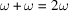) and SFG (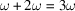) in different crystals  (pp. 206-207) | html | pdf |
• 1.7.3.3.3.2. SHG () and SFG () in the same crystal  (p. 207) | html | pdf |
• 1.7.3.3.3.3. Direct THG (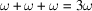)  (p. 207) | html | pdf |
• 1.7.3.3.4. Sum-frequency generation (SFG)  (pp. 207-208) | html | pdf |
• 1.7.3.3.4.1. SFG (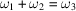) with undepletion atand(p. 208) | html | pdf |
• 1.7.3.3.4.2. SFG () with undepletion at(p. 208) | html | pdf |
• 1.7.3.3.5. Difference-frequency generation (DFG)  (pp. 208-212) | html | pdf |
• 1.7.3.3.5.1. DFG (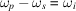) with undepletion atand(p. 208) | html | pdf |
• 1.7.3.3.5.2. DFG (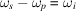) with undepletion at(p. 208) | html | pdf |
• 1.7.3.3.5.3. DFG () with undepletion at– optical parametric amplification (OPA), optical parametric oscillation (OPO)  (pp. 208-212) | html | pdf |
• 1.7.4. Determination of basic nonlinear parameters  (pp. 212-214) | html | pdf |
• 1.7.4.1. Phase-matching directions and associated acceptance bandwidths  (p. 212) | html | pdf |
• 1.7.4.2. Nonlinear coefficients  (pp. 212-214) | html | pdf |
• 1.7.4.2.1. Non-phase-matched interaction method  (pp. 212-214) | html | pdf |
• 1.7.4.2.2. Phase-matched interaction method  (p. 214) | html | pdf |
• 1.7.5. The main nonlinear crystals  (pp. 214-216) | html | pdf |
• 1.7.6. Glossary  (p. 216) | html | pdf |
• References | html | pdf |
• Figures
• Fig. 1.7.3.1. Field vectors of a plane wave propagating in an anisotropic medium  (p. 183) | html | pdf |
• Fig. 1.7.3.2. Index surfaces of the negative and positive uniaxial classes  (p. 186) | html | pdf |
• Fig. 1.7.3.3. Index surfaces of the negative and positive biaxial classes  (p. 186) | html | pdf |
• Fig. 1.7.3.4. Index surface sections in a plane containing the optic axis z of a negative uniaxial crystal  (p. 189) | html | pdf |
• Fig. 1.7.3.5. Stereographic projection on the optical frame of the possible loci of phase-matching directions in the principal planes of a biaxial crystal  (p. 193) | html | pdf |
• Fig. 1.7.3.6. Schematic configurations for second harmonic generation  (p. 196) | html | pdf |
• Fig. 1.7.3.7. Spatial growth evolution of second harmonic conversion efficiency  (p. 198) | html | pdf |
• Fig. 1.7.3.8. Conversion efficiency evolution as a function of ξ for a given crystal length  (p. 198) | html | pdf |
• Fig. 1.7.3.9. Beam separation in the particular case of type-II ( oeo ) SHG out of the xy plane of a positive uniaxial crystal or in the xz and yz planes of a positive biaxial crystal  (p. 201) | html | pdf |
• Fig. 1.7.3.10. Twin-crystal device allowing walk-off compensation for a direction of propagation θ PM in the yz plane of a positive uniaxial crystal  (p. 201) | html | pdf |
• Fig. 1.7.3.11. Comparison between ( a ) collinear and ( b ) special non-collinear phase matching for ( oeo ) type-II SHG  (p. 202) | html | pdf |
• Fig. 1.7.3.12. Position f opt of the beam waist for different values of walk-off angles and, leading to an optimum SHG conversion efficiency  (p. 203) | html | pdf |
• Fig. 1.7.3.13. Optimum walk-off function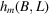as a function offor various values of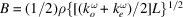(p. 203) | html | pdf |
• Fig. 1.7.3.14. Type-I and -II conversion efficiencies calculated as a function offor different typical walk-off angles ρ  (p. 204) | html | pdf |
• Fig. 1.7.3.15. Schematic SHG conversion efficiency for different situations of pump depletion and dephasing  (p. 205) | html | pdf |
• Fig. 1.7.3.16. Configurations for third harmonic generation  (p. 206) | html | pdf |
• Fig. 1.7.3.17. Frequency up-conversion process(p. 207) | html | pdf |
• Fig. 1.7.3.18. Schematic OPO configurations  (p. 209) | html | pdf |
• Fig. 1.7.3.19. Calculated angular tuning curves  (p. 211) | html | pdf |
• Fig. 1.7.3.20. Calculated pump wavelength tuning curve  (p. 211) | html | pdf |
• Fig. 1.7.4.1. ( a ) The Maker-fringes technique; ( b ) the wedge-fringes technique  (p. 214) | html | pdf |
• Tables
• Table 1.7.2.1. The most common nonlinear effects and the corresponding susceptibility tensors in the frequency domain  (p. 181) | html | pdf |
• Table 1.7.2.2. Nonzero χ (2) coefficients and equalities between them in the general case  (p. 182) | html | pdf |
• Table 1.7.2.3. Nonzero χ (2) coefficients and equalities between them under the Kleinman symmetry assumption  (p. 183) | html | pdf |
• Table 1.7.2.4. Nonzero χ (3) coefficients and equalities between them in the general case  (p. 184) | html | pdf |
• Table 1.7.2.5. Nonzero χ (3) coefficients and equalities between them under the Kleinman symmetry assumption  (p. 185) | html | pdf |
• Table 1.7.3.1. Correspondence between the phase-matching relations, the configurations of polarization and the types according to the sum- and difference-frequency generation processes SFG (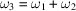), DFG (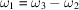) and DFG (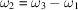)  (p. 188) | html | pdf |
• Table 1.7.3.2. Correspondence between the phase-matching relations, the configurations of polarization and the types according to SFG (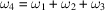), DFG (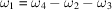), DFG () and DFG (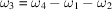) (Boulanger et al. , 1993)  (p. 189) | html | pdf |
• Table 1.7.3.3. Classes of refractive-index inequalities for collinear phase matching of three-wave interactions in positive and negative uniaxial crystals  (p. 190) | html | pdf |
• Table 1.7.3.4. Classes of refractive-index inequalities for collinear phase matching of four-wave interactions in positive (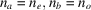) and negative (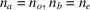) uniaxial crystals with(p. 190) | html | pdf |
• Table 1.7.3.5. Refractive-index conditions that determine collinear phase-matching loci in the principal planes of positive and negative biaxial crystals for three-wave SFG  (p. 191) | html | pdf |
• Table 1.7.3.6. Refractive-index conditions that determine collinear phase-matching loci in the principal planes of positive and negative biaxial crystals for four-wave SFG  (pp. 192-193) | html | pdf |
• Table 1.7.3.7. Matrix representations of the ( oee ) and ( eoo ) field tensors of the uniaxial class and of the biaxial class in the principal planes xz and yz , with(Boulanger & Marnier, 1991)  (p. 195) | html | pdf |
• Table 1.7.3.8. Matrix representations of the ( oeee ), ( eooo ) and ( ooee ) field tensors of the uniaxial class and of the biaxial class in the principal planes xz and yz , with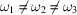(Boulanger et al. , 1993)  (p. 195) | html | pdf |
• Table 1.7.3.9. Field-tensor components specifically nil in the principal planes of uniaxial and biaxial crystals for three-wave and four-wave interactions  (p. 196) | html | pdf |
• Table 1.7.5.1. Mineral nonlinear crystals  (pp. 213-214) | html | pdf |
• Table 1.7.5.2. Organic and organo-mineral crystals  (pp. 215-216) | html | pdf |